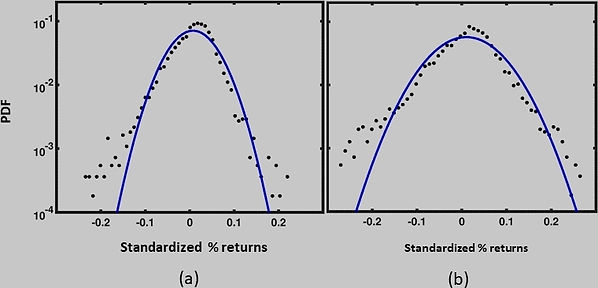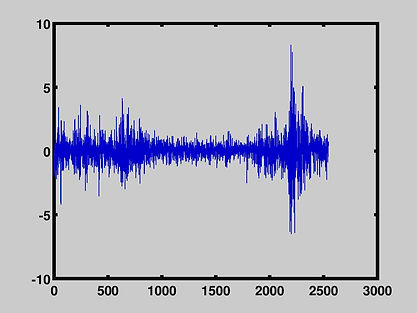top of page

# Nonextensive Statistical Mechanics

What is non-extensive statistical mechanics? Before answering this let us first distinguish between Extensive  and Non-extensive systems.

### Extensive Systems

In these systems, the microscopic entities essentially follow a random walk. The extensive variables such as Internal energy,  Entropy,  Number of particles etc of the system scale with the size of the system, ie, if you have two systems, the extensive variables for the combined system are the sum of the variables from each system. It can also be shown, that the equilibrium energy distributions are Gaussian (bell curve) .

Efficient markets are extensive systems. If this is so, the stock market returns should show the following characteristics.

a) No volatility clustering

The data has no structure. (In this model there cannot be any crashes, bubbles ----).

b) Short relaxation time (The auto correlation falls off exponentially).

c) The distribution of returns is Gaussian. Following figure shows the comparison of the distributions of SP 500 and Nasdaq monthly returns with the corresponding Gaussian distribution. They don't agree.

### ​

Unlike extensive systems,  the microscopic entities in non-extensive systems  have a long range interaction and hence do not follow a random walk. Because of ths interaction, the variables (Internal energy,  Entropy, Number of particles etc ) are not additive and the energy distributions are non-Gaussian. An example of non-extensive system is a fractal system.

Real stock market returns behave more like non-extensive systems.

They show the following characteristics

a) Volatility clustering

The data shows structures.(bubbles, crashes --- can happen).

b) Longer relaxation time (The auto correlation shows a power law behavior).

c) The distributions of returns are non-Gaussian. They have fat tails.

Several studies indicate that the issues connected with turbulent/chaotic and non-linear systems (non-extensive) such as present day stock markets can be addressed using non-extensive statistics developed by Tsallis, which is a generalization of normal statistics to non-extensive systems. These methods were originally proposed to study classical and quantum chaos, physical systems far from equilibrium such as turbulent systems, and long range interacting Hamiltonian systems.  However, in the last several years, there has been considerable interest in applying these methods to analyze financial market dynamics as well. Such applications fall into the category of econophysics.

Our own research shows that the real stock data characteristics are reasonably well reproduced by Tsallis statistics.  For details see publications 3 and 4 in Research.bottom of page